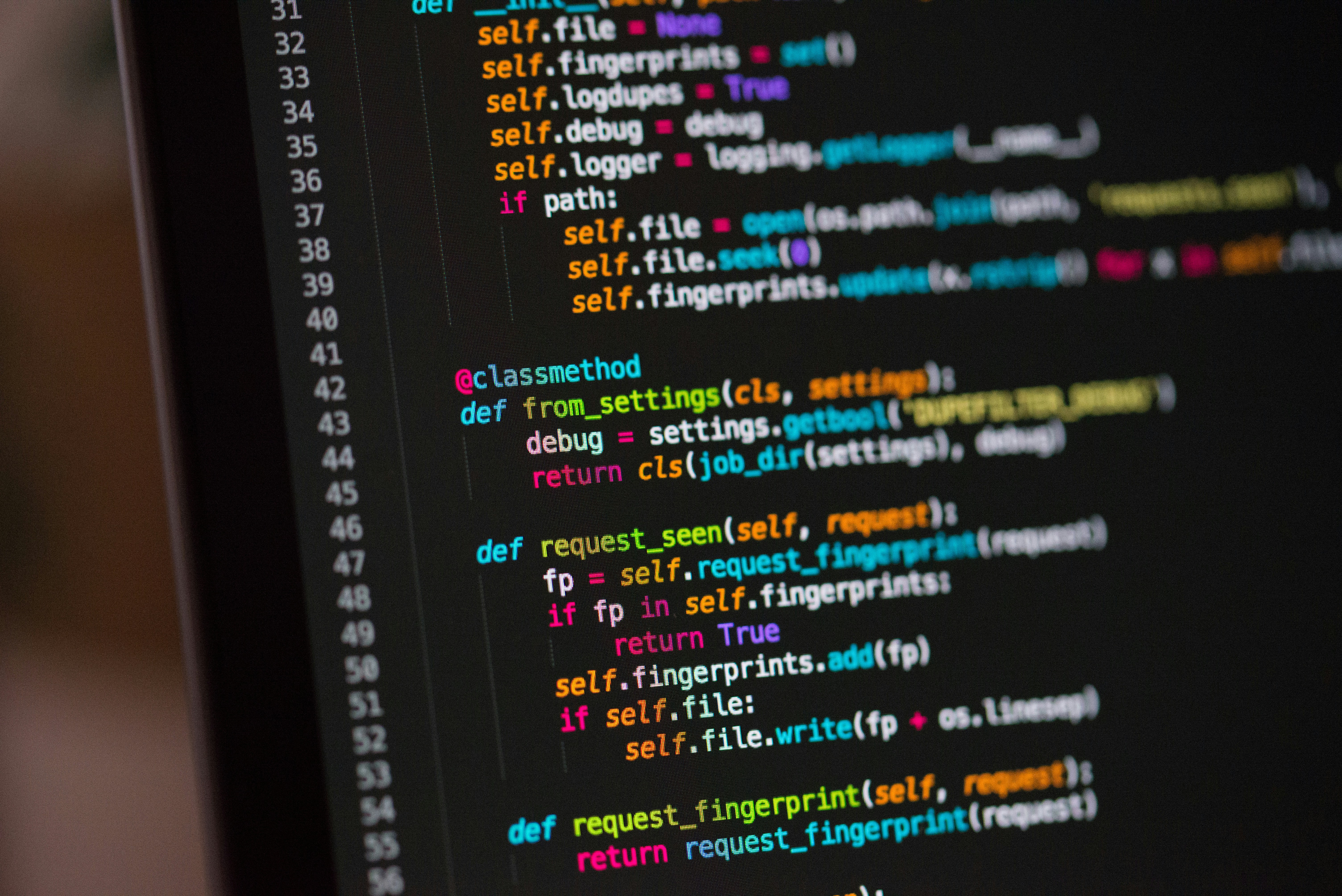Photo by Chris Ried / Unsplash

# Part II: Implementing RSA in Python from Scratch

Programming Aug 21, 2021

In the last article RSA key generation and integer encryption were explained and implemented. This is good for demonstrating how the algorithm works, but it is not really usable if you want to exchange encrypted messages with someone. To be usable, it needs big random prime generation and text encryption, so those will be explained in this part.

## Random prime number generation

To maka sure nobody can factorize your `n` and find the decryption key, it is recommended to use 2048-bit values for `n`. This means that the prime numbers should be around 1024 bits long. The method is to randomly generate numbers until a prime is found. Since the numbers generated are very big, heuristic tests such as Selfridge's conjure are preferable over classical methods which are a lot slower.

The PWS conjure states:
If a number `p` gives reminders 3 and 7 `(mod 10)` (equivalent of saying that `p` is odd and `±2 (mod 5)`), then it is prime if the following conditions hold:

• `2^(p - 1) ≡ 1 (mod p)`
• `(p + 1)-th Fibonacci number ≡ 0 (mod p)`

Fast exponentiation with modulo was already implemented in the last article, so only Fibonacci number calculator with modulo needs to be implemented.

Fibonacci number calculation

Let `f` be a function that calculates next Fibonacci number, given last 2.
`f(a, b) = (b, a + b)`
To calculate 4th Fibonacci number you'd do `f(f(1, 1))`.
To calculate 10th you'd do `f(f(f(f(f(f(f(f(1, 1))))))))`, or simplified, `(f^8)(1, 1)`.
To calculate `n`-th Fibonacci number you'd do `(f^(n - 2))(1, 1)`.
Since `f` is a linear function, it can be represent by a matrix.

``````[ 0 1 ]
[ 1 1 ]
``````

(Matrix multiplication won't be explained in this article so in case you don't know it, it is recommended that you look it up before continuing)

Using matrices, you can calculate the n-th Fibonacci number like this:

``````[ 1 ] [ 0 1 ]^n  = [ (n - 1)-th Fibonacci number ]
[ 1 ] [ 1 1 ]    = [       n-th Fibonacci number ]
``````

Matrix multiplication is associative (`(M1 * M2) * M3 = M1 * (M2 * M3)`) so you can apply the same optimization as in `modpow` from last article.

``````# matrix multiplication
def sqmatrixmul(m1, m2, w, mod):
mr = [[0 for j in range(w)] for i in range(w)]
for i in range(w):
for j in range(w):
for k in range(w):
mr[i][j] =(mr[i][j]+m1[i][k]*m2[k][j])%mod
return mr

# fibonacci calculator
def fib(x, mod):
if x < 3: return 1
x -= 2
# find length of e in bits
tst = 1
siz = 0
while x >= tst:
tst <<= 1
siz += 1
siz -= 1
# calculate the matrix
fm = [
# function matrix
[0, 1],
[1, 1]
]
rm = [
# result matrix
# (identity)
[1, 0],
[0, 1]
]
for i in range(siz, -1, -1):
rm = sqmatrixmul(rm, rm, 2, mod)
if (x >> i) & 1:
rm = sqmatrixmul(rm, fm, 2, mod)

# second row of resulting vector is result
return (rm + rm) % mod
``````

The prime number generation can then be implemented like this:

``````def genprime(siz):
while True:
num = (1 << (siz - 1)) + secrets.randbits(siz - 1) - 10;
# num must be 3 or 7 (mod 10)
num -= num % 10
num += 3 # 3 (mod 10)
# heuristic test
if modpow(2, num - 1, num) ==1 and fib(num + 1, num) ==0:
return num
num += 5 # 7 (mod 10)
# heuristic test
if modpow(2, num - 1, num) ==1 and fib(num + 1, num) ==0:
return num
``````

NOTE: Instead of using `random` module, here is used `secrets`. `random` is good for generating statistically random numbers, but it can be predicted and is therefore less preferable in cryptography.

## Plaintext encryption and decryption

Since everything is calculated modulo `n`, representing the whole byte array as a single number and encrypting it would result in data loss, so you should instead split the data up into subsequences and encrypting those separately. The longer the subsequences are, the smaller are chances of the message being guessed by randomly generating sequences until their ciphertexts match. Since `n` is 2048-bit, the biggest the sequence can be is 256 bytes (256 * 8 = 2048).

The message length will not necessarily be divisible by 256, so the last block will have to be padded by trailing 0x00s. Because of this, it is also good to store plaintext length together with ciphertext or inside the plaintext itself in case binary files are exchanged.

``````def encrypt_bytes(data, key):
data = bytearray(data)
cdata = bytearray()
for i in range(0, len(data), 256):
# read 256 bytes and store as long
# to m
m = 0
for j in range(256):
if i + j < len(data):
m = (m << 8) + data[i + j]
else:
m <<= 8
# encrypt m
c = modpow(m, key, key)
# store c into cdata
for j in range(255, -1, -1):
cdata.append((c >> (j * 8)) & 255)
return bytes(cdata)

# both functions are essencially the same,
# the only difference is in which key you use
decrypt_bytes = encrypt_bytes
``````

## Conclusion

With the code presented here and in the last article, you can make a working RSA encryption & decryption application. However, it still lacks protection from side-channel attacks.

All code has been published to this repository, together with a small script that let's you encrypt and decrypt text files (it is not suitable for binary files because of the aforementioned issue with padding with zeros).

I hope this article helped you better understand the technology that allows us to securely exchange sensitive information over internet.